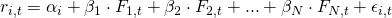# Factor models

Instead of using mean-variance portfolio optimization, investors can opt for an alternative approach based on factor models. These models relate the return of a security to a number of (risk) factors. Generally, they have the following structurewhere ri,t is security i‘s return at time t, F1,t, F2,t, to FN,t are the factors, and β1, β2 to βN are the factor loadings. The factors included in a factor model can be macroeconomic variables, stock indices, or other portfolios. The factor loadings measure the sensitivity of the security to changes in the factor. For example, suppose that one of the factors is the return on the S&P500. Now, imagine we estimate the model and obtain a β equal 2. This means that, if the S&P500 increase by 1%, then the security tends to go up by 2%, on average.

The equation also contains a so-called error term (ε). This error term is necessary to be able to estimate the (regression) model. However, we can also give an economic interpretation to this parameter. In particular, the error term represents security-specific news that becomes available to investors.

The coefficient that we are actually most interested in, is the constant in the factor model. We’ve denoted it using the greek symbol α and investors will also typically refer to it as the security’s ‘alpha’ . α measures the return of a security that cannot be explained by exposure to the factors in the model or firm-specific news. As such, it measures the security’s excess return.

## Portfolio construction

How can factor models be used in the context of portfolio construction?

Basically, we want to achieve the following objectives:

• maximize the (excess) return of the portfolio, i.e. maximize the alpha of the portfolio
• minimize volatility of the portfolio that is due to stock market fluctuations, i.e. minimize the beta of the portfolio
• minimize the impact of firm-specific news, i.e. being sufficiently diversified

Note that the approach only makes sense if the investors can correctly determine which stocks have a positive alpha. Thus, the investors should have stock-picking skills.

One way of solving the above problem was proposed by Treynor and Black. The approach is referred to as the Treynor-Black model.

## Summary

Factor models can be used to identify potentially interesting securities that can be added to a portfolio. In addition, the results can be used to construct better portfolios.